# Class 10 Maths Extra Questions for Linear equation

Given below are the Class 10 Maths Extra Questions And Important Questions for Linear equations in two variables
a. Concepts questions
b. True and False
c. Multiple choice questions

## Concept Questions

Question 1..
Which of these equation have (i) Unique solution (ii) Infinite solutions (iii) no solutions
1. $152x-378y=-74$ , $-378x +152y=-604$
2. $x+2y =10$ , $3x+6y=30$
3. $3x+4y=6$ , $12x+16x=30$
4. $7x-11y=53$ ,$19x -17y=456$
5. $x=7$,$y=-2$
6. $ax+by=a-b$ , $bx-ay=a+b$
7. $2x+3y=0$, $124x+13y=0$
8. $y=11$ ,$y =-11$
Solution
 Condition Algebraic interpretation $\frac{a_{1}}{a_{2}}\neq \frac{b_{1}}{b_{2}}$ One unique solution only. $\frac{a_{1}}{a_{2}}= \frac{b_{1}}{b_{2}}=\frac{c_{1}}{c_{2}}$ Infinite solution. $\frac{a_{1}}{a_{2}}= \frac{b_{1}}{b_{2}}\neq \frac{c_{1}}{c_{2}}$ No solution
One unique solution: (a), (d), (e), (f), (d)
Infinite solution :( b)
No solution:  (c), (h)

Question 2.
True or False statement
a. Line $4x+5y=0$ and $11x+17y=0$ both passes through origin
b. Pair of lines $117x+14y=30$ , $65x+11y=19$ are consistent and have a unique solution
c. There are infinite solution for equation $17x+12y=30$
d. $x=0$ ,$y=0$ has one unique solution
e. Lines represented by $x-y=0$ and $x+y=0$ are perpendicular to each other
f. $2x+6y=12$ and $8x+24y=65$ are consistent pair of equation
g. $x+6y=12$ and $4x+24y=64$ are inconsistent pair of equation
Solution
1. True
2. True
3. True
4. True
5. True
6. False
7. True

## Multiple choice Questions

Question 3.
find the value of p for which the linear pair has infinite solution
$12x+14y=0$
$36x+py=0$
a. 14
b. 28
c. 56
d. 42
Solution (d)
For infinite solution
$\frac{a_{1}}{a_{2}}= \frac{b_{1}}{b_{2}}$
So $\frac {12}{36}=\frac {14}{p}$ => p=42

Question 4.
There are 10 students in XII class. Some are maths and some bio student. The no of bio students are 4 more then math’s students. Find the no of math’s and bio students
a. 1,9
b. 4,6
c. 2,8
d. 3,7
Solution (d)
Let x be math’s students
y be bio students
Then
$x+y=10$
$y=x+4$
Solving these linear pair through any method we get
x=3 and y=7

Question 5.
which of the below pair are consistent pair?
a. $x-3y=3$ , $3x-9y=2$
b. $51x +68y=110$ ,$3x+4y=99$
c. $2x+3y=10$ , $9x+11y=12$
d. None of these
Solution c
For consistent pair
$\frac{a_{1}}{a_{2}}= \frac{b_{1}}{b_{2}}$
Or
$\frac{a_{1}}{a_{2}}= \frac{b_{1}}{b_{2}}=\frac{c_{1}}{c_{2}}$
Analyzing all of them ,we get c as the answer

Question 6.
There are two numbers. Two conditions are there for them
(i) Sum of these two numbers are 100
(ii) One number is four time another number.
What are these numbers?
a. 20,80
b. 30,70
c. 40,60
d. 25,75
Solution (a)
Let x and y are the number
$x+y=100$
$y=4x$
Solving them we get x=20 and y=80

Question 7.
The number of solution of the linear pair
$x+37y=123$
$21x-41y=125$
a. No solution
b. One solution
c. infinite many
d. None of these
Solution (d)

Question 8.
The sum of a 2 digit number and number obtained by reversing the order of the digits is 99. If the digits of the number differ by 3. Find the number
Solution  63 or 36

Question 9.
Rajdhani train covered the distance between Lucknow and Delhi  at a uniform speed. It is observed that  if rajdhani would have run slower by 10 km/hr,it would have taken 3 hours more to reach the destination and if rajdhani would have run faster by 10 km/hr,it would have taken 2 hours less. Find the distance Lucknow and Delhi?
Solution
Let x be the speed and t be the original timing ,then distance between Lucknow and Delhi
$Distance =speed \times time =xy$
Now from first observation
$xy=(x-10)(y+3)$ => $3x-10y-30=0$
From second observation
$xy=(x+10)(y-2)$ => $2x-10y+20=0$
Solving both we get
x=50km/hr
y=12hours
So distance between Lucknow and Delhi=$50 \times 12=600$ Km

Question 10.
Using substitution method solve the below equation
a. $x-2y+300=0$, $6x-y-70=0$
b. $5x-y=5, 3x-y=3$
Solution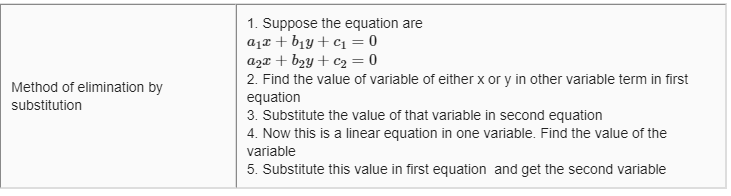a. From Ist equation
$x=2y-300$
Substituting this in second equation
$6(2y-300)- y-70=0$ => $y=170$
Putting this Ist equation
$x=40$
b. Similarly,this can be solved. Answer is ( 1,0)

Question 11.
Using elimination method ,solve the following
a. $x+y-40=0$ ,$7x+3y=180$
b. $x+10y =68$ , $x+15y=98$
Solution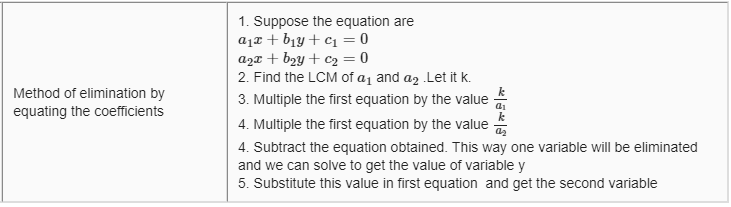a. $x+y-40=0$ ---(1)
$7x+3y=180$ ---(2)
Multiplying equation (1) by 7
$7x+7y-280=0$ ---(3)
Subtracting equation 2 from equation 7
$7x+7y-280=0$
$7x+3y=180$
We get
$4y=100$ => $y=25$
Substituting this in (1) ,we get $x=15$
b. ( 8,6)

Question 12.
Solve the below linear equation using cross-multiplication method
a. $(a-b)x + (a=b) y=a^2-2ab-b^2$ , $(a+b)(x+y)=a^2+ b^2$
b. $x+y=5$ ,$2x-3y=4$
Solution
 3 Cross Multiplication method 1. Suppose the equation are $a_1x+b_1y+ c_1=0$ $a_2x +b_2y+c_2=0$ 2. This can be written as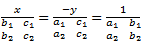3. This can be written as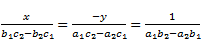4. Value of x and y can be find using the x => first and last expression y=> second and last expression
a. The equation can be written as
$(a-b)x + (a=b) y=a^2-2ab-b^2$
$(a+b)(x+y)=a^2+ b^2$
By cross multiplication we have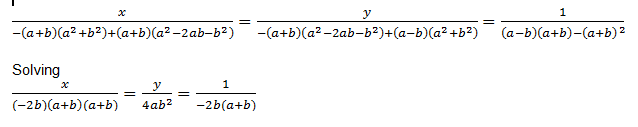$x=a+b$, $y=-2ab(a+b)^{-1}$
b. (19/5,6/5)

Question 13.
For what value of k, the following pair of linear equations has infinitely many solutions?
i. $kx + 4y - (k + 8) = 0$
$4x + ky + 4 = 0$
ii. $2x + 3y = 4$
$(k + 2)x + 6y = 3k + 2$

Question 14
For what value of k, will the following system of equations have no solutions?
i. $(3k + 1) x + 3y = 2$
$(k^2 + 1) x + (k - 2) y = 5$
ii. $3x + y = 1$
$(2k - 1) x + (k - 1) y = 2k + 1$
iii. $2x + ky = 11$
$5x -7y = 5$

Question 15.
For what value of k, does the pair of equations given below has a unique solution?
$2x + ky = 6$
$4x + 6y = 0$

Question 16.
Find the value of k for which the following system of linear equations has infinite solutions:
i. $x + (k + 1) y = 5$
$(k + 1) x + 9y = 8k - 1$
ii. $8x + 5y = 9$
$kx + 10y = 18$
iii. $2x + 3y = k$
$(k - 1) x + (k + 2) y = 3k$

Question 17
For what value of α, the system of equations
$\alpha x + 3y = \alpha - 3$
$12x + \alpha y = \alpha$
will have no solution?
-6

Question 18.
Find the value of k for which the system
$kx + 2y = 5$
$3x + y = 1$
has (i) a unique solution, and (ii) no solution.
k ≠6 , k=6

Question 19.
Find the value of k for which the system
$2x + ky = 1$
$3x - 5y = 7$
has (i) a unique solution, and (ii) no solution.
k ≠ -10/3 , k=10/3

Question 20
find the value of a and b for which the following system of equations has infinitely many solutions:
$2x - (2a + 5) y = 5$
$(2b + 1) x - 9y = 15$
(-1, 5/2)
Question 21.
Find the value of a for which the following system of equations has infinitely many solutions:
$2x + 3y - 7 = 0$
$(a - 1) x + (a + 1) y = (3a - 1)$
a=5

Question 22.
Replace p by an appropriate number so that the following system of equations has a unique solution.
$2x + 3y = 5$
$px +9y = 12$

## Summary

This pair of linear equations in two variables class 10 extra questions with solutions is prepared keeping in mind the latest syllabus of CBSE . This has been designed in a way to improve the academic performance of the students. If you find mistakes , please do provide the feedback on the mail.Go back to Class 10 Main Page using below links

### Practice Question

Question 1 What is $1 - \sqrt {3}$ ?
A) Non terminating repeating
B) Non terminating non repeating
C) Terminating
D) None of the above
Question 2 The volume of the largest right circular cone that can be cut out from a cube of edge 4.2 cm is?
A) 19.4 cm3
B) 12 cm3
C) 78.6 cm3
D) 58.2 cm3
Question 3 The sum of the first three terms of an AP is 33. If the product of the first and the third term exceeds the second term by 29, the AP is ?
A) 2 ,21,11
B) 1,10,19
C) -1 ,8,17
D) 2 ,11,20